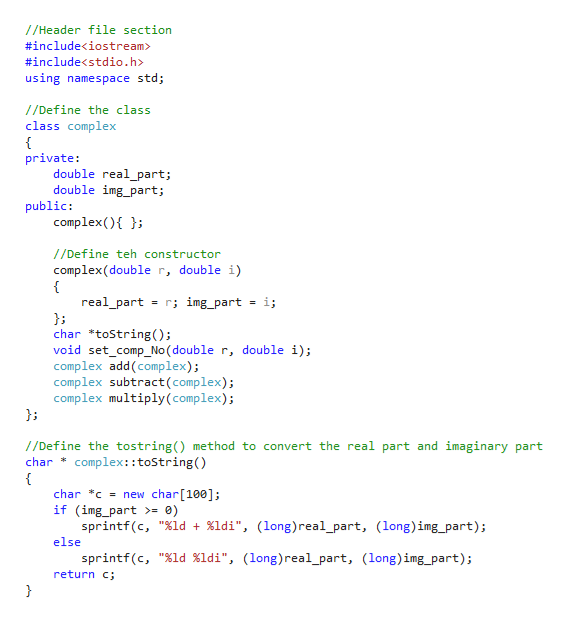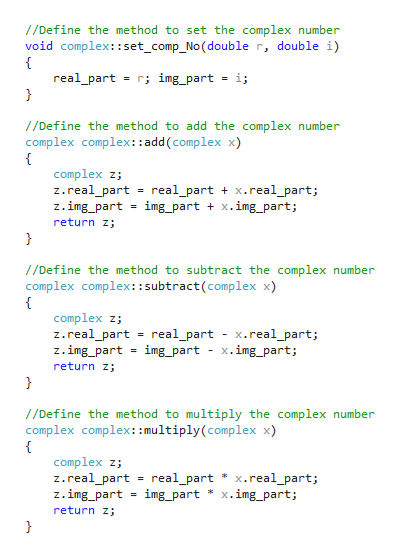# Using C++ 11Create a class called Complex for performing arithmetic with complex numbers. Write a program to test your class. Complex numbers have the form:realPart + imaginaryPart * iWhere i is  − 1Use double variables to represent the private data of the class. Provide a constructor that enables an object of this class to be initialized when it is declared. The constructor should contain default values in case no initializers are provided. Provide public member functions that perform the following tasks:a) Adding two complex numbers: The real parts added together and the imaginary parts are added together. You should pass two objects to your function.b) Subtracting two complex numbers: The real parts subtracted together and the imaginary parts are subtracted together. You should pass two objects to your function.c) Printing Complex numbers in the form (a , b), where a is the real part and b is the imaginary part.(Do not use operator overloading for this assignment.)

Question

Using C++ 11

Create a class called Complex for performing arithmetic with complex numbers. Write a program to test your class. Complex numbers have the form:

realPart + imaginaryPart * i

Where is  − 1

Use double variables to represent the private data of the class. Provide a constructor that enables an object of this class to be initialized when it is declared. The constructor should contain default values in case no initializers are provided. Provide public member functions that perform the following tasks:

1. a) Adding two complex numbers: The real parts added together and the imaginary parts are added together. You should pass two objects to your function.
2. b) Subtracting two complex numbers: The real parts subtracted together and the imaginary parts are subtracted together. You should pass two objects to your function.
3. c) Printing Complex numbers in the form (a , b), where a is the real part and b is the imaginary part.

check_circleExpert Solution
Step 1

This program demonstrates the complex numbers for performing arithmetic.

Program:

#include<iostream>
#include<stdio.h>
using namespace std;
//Define the class
class complex
{
private:
double real_part;
double img_part;
public:
complex(){ };

//Define teh constructor
complex(double r, double i)
{
real_part = r; img_part = i;
};
char *toString();
void set_comp_No(double r, double i);
complex subtract(complex);
complex multiply(complex);
};
//Define the tostring() method to convert the real part and imaginary part
char * complex::toString()
{
char *c = new char;
if (img_part >= 0)
sprintf(c, "%ld + %ldi", (long)real_part, (long)img_part);
else
sprintf(c, "%ld %ldi", (long)real_part, (long)img_part);
return c;
}
//Define the method to set the complex number
void complex::set_comp_No(double r, double i)
{
real_part = r; img_part = i;
}
//Define the method to add the complex number
{
complex z;
z.real_part = real_part + x.real_part;
z.img_part = img_part + x.img_part;
return z;
}
//Define the method to subtract the complex number
complex complex::subtract(complex x)
{
complex z;
z.real_part = real_part - x.real_part;
z.img_part = img_part - x.img_part;
return z;
}
//Define the method to multiply the complex number
complex complex::multiply(complex x)
{
complex z;
z.real_part = real_part * x.real_part;
z.img_part = img_part * x.img_part;
return z;
}
//Main function definition
int main()
{
//Pass the arguments
complex c1(2.0, 5.0), c2;
complex a, s, m;
c2.set_comp_No(3.0, 4.0);
//Print the complex numbers
cout << "Complex number-1 : " << c1.toString() << endl;
cout << "Complex number-2 : " << c2.toString() << endl;
cout << "Addition of complex numbers c1 + c2 = " << a.toString() << endl;
//Call the subtract() function to subtract the complex number
s = c1.subtract(c2);
cout << "Subtraction of complex numbers c1 - c2 = " << s.toString() << endl;
//Call the multiply() function to multiply the complex number
m = c1.multiply(c2);
cout << "Multiplication of complex numbers c1 * c2 = " << m.toString() << endl;
return 0;
}

Step 2

Screenshot#1:help_outlineImage Transcriptionclose/Header file section #include #include using namespace std; /Define the class class complex private: double realpart; double img_part; public: complex() //Define teh constructor complex(double r, double i) real_part r; img_part i; char toString(); void set_comp_No ( double r, double i); complex add(complex) complex subtract(complex); complex multiply ( complex) ; //Define the tostring() method to convert the real part and imaginary part complex::toString () char char cnew char; if (img_part >= 0) sprintf(c, "%ld %ldi", (long)real_part, (long) img_part); else sprintf(c, "%ld %ldi", (long)real_part, (long) img_part); return c fullscreen
Step 3

Screenshot #2:

...help_outlineImage Transcriptionclose/Define the method to set the complex number void complex::set_comp_No (double r, double i) = r; img_part = i; real_part } //Define the method to add the complex number complex complex:add (complex x) complex z z.real_part z.img_part return z real_partx.real_part; img_part x.img_part; } /Define the method to subtract the complex number complex complex::subtract(complex x) complex z z.real_part z.img_part return z } real_part - x.real_part; img_part = x.img_part; //Define the method to multiply the complex number complex complex: :multiply (complex x) complex z; z.real_part = real_part * x.real_part; z.img_part return z img_part x.img_part; fullscreen

### Want to see the full answer?

See Solution

#### Want to see this answer and more?

Solutions are written by subject experts who are available 24/7. Questions are typically answered within 1 hour*

See Solution
*Response times may vary by subject and question
Tagged in

### Object Oriented Programming